# Inner Product Question

• Onezimo Cardoso

## Homework Statement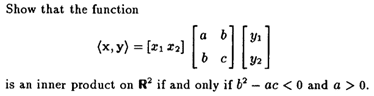## Homework Equations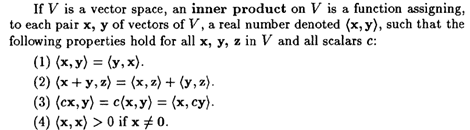## The Attempt at a Solution

[/B]
I could show that the first of the three properties are valid for any value of a,b,c but I couldn’t find a way to show the forth one.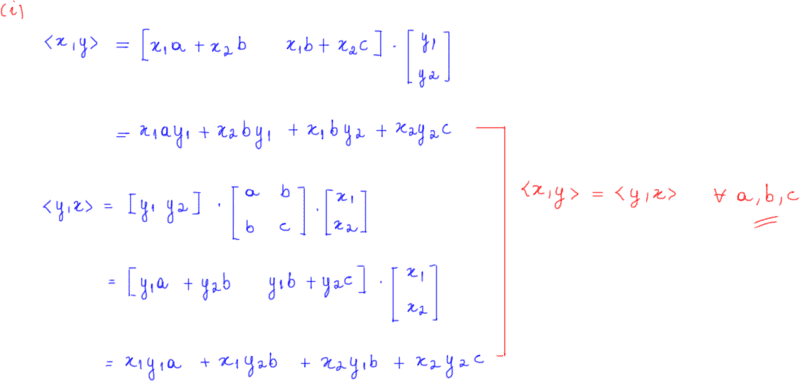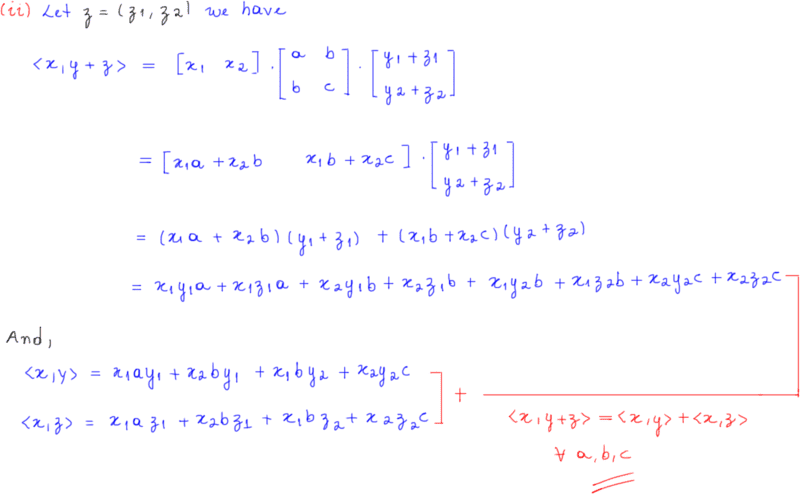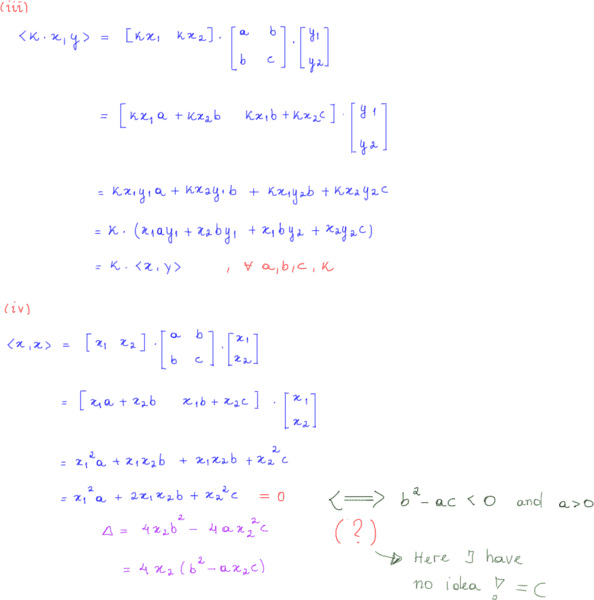#### Attachments

Onezimo Cardoso said:

## Homework Statement

View attachment 207920

## Homework Equations

View attachment 207921

## The Attempt at a Solution

[/B]
I could show that the first of the three properties are valid for any value of a,b,c but I couldn’t find a way to show the forth one.

Hint: if ##{\bf x} = (x_1,0)##, it is easy to show that ##\langle {\bf x,x} \rangle > 0## for any ##x_1 \neq 0##. Also: for ##x_2 \neq 0## we have ##(x_1,x_2) = x_2(x_1/x_2,1)##, so ##\langle {\bf x,x} \rangle = x_2^2 \langle {\bf u,u} \rangle##, where ##{\bf u} = (x_1/x_2,1) \equiv (t,1)##.

•Onezimo Cardoso
•Onezimo Cardoso
are you allowed to use spectral theory here?

##
\begin{bmatrix}
a & b\\
b & c
\end{bmatrix}##

is real symmetric. If the determinant is positive (one of your conditions in the iff) and the trace is positive (implied by ##a \gt 0##... why?) then it this tells you...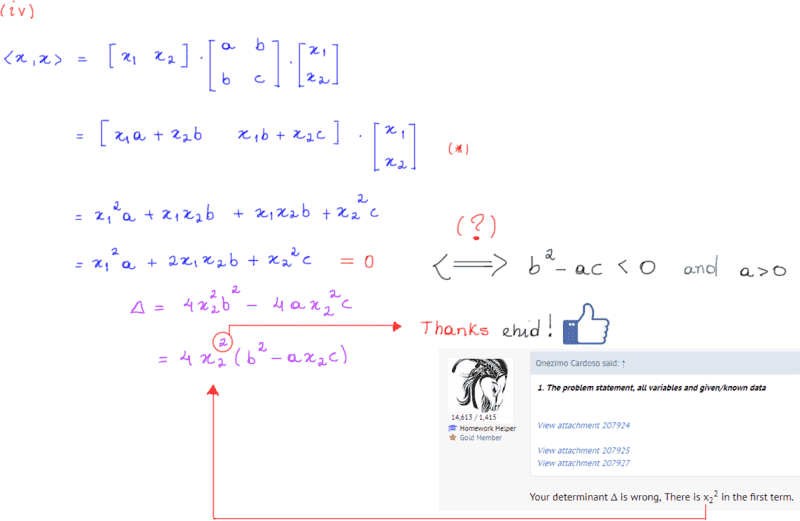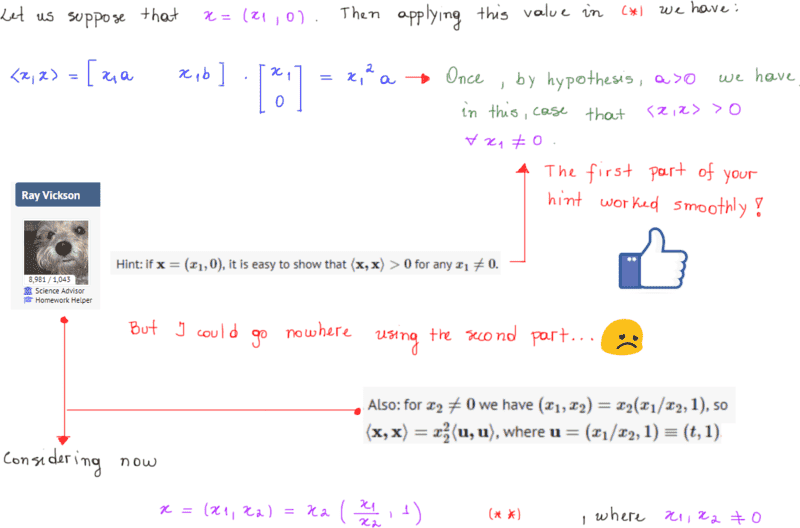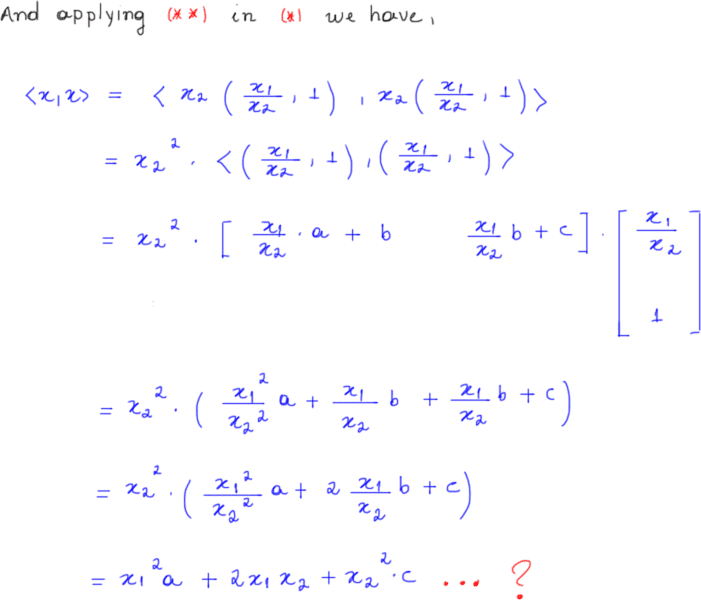#### Attachments

Onezimo Cardoso said:

## The Attempt at a Solution

[/B]
I could show that the first of the three properties are valid for any value of a,b,c but I couldn’t find a way to show the forth one.